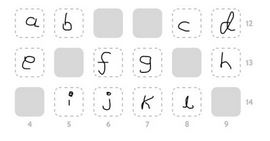# $12/15/2020$ - Daily Challenge Solution$\underline{\text{Equations}}$

\begin{aligned} a + e &= 4 \\ b + i &= 5 \\ f + j &= 6 \\ g + k &= 7 \\ c + l &= 8 \\ d + h &= 9 \\ a + b + c + d &= 12 \\ e + f + g + h &= 13 \\ i + j + k + l &= 14 \end{aligned}

$\underline{\text{Brute-Force Case Work}}$

$a$ can be either one of $1, 2$ and $3$.

$d$ can be either one of $3, 4, 5$ and $6$.

Thus, their possible sums are $4, 5, 6, 7, 8$ and $9$.

$b$ can be either one of $1, 2, 3$ and $4$.

$c$ can be either one of $2, 3, 5$ and $6$.

Thus, their possible sums are $3, 4, 5, 6, 7, 8, 9$ and $10$.

(Let $|$ be the same as 'or'.)

So, $a + b + c + d$ is the same as $[4 | 5 | 6 | 7 | 8 | 9] + [3 | 4 | 5 | 6 | 7 | 8 | 9 | 10]$

The only pairs here that make $12$ are $[3 + 9], [4 + 8], [5 + 7] \text{ and } [6 + 6]$

Meh, this is getting nowhere...Note by A Former Brilliant Member
7 months, 1 week ago

This discussion board is a place to discuss our Daily Challenges and the math and science related to those challenges. Explanations are more than just a solution — they should explain the steps and thinking strategies that you used to obtain the solution. Comments should further the discussion of math and science.

When posting on Brilliant:

• Use the emojis to react to an explanation, whether you're congratulating a job well done , or just really confused .
• Ask specific questions about the challenge or the steps in somebody's explanation. Well-posed questions can add a lot to the discussion, but posting "I don't understand!" doesn't help anyone.
• Try to contribute something new to the discussion, whether it is an extension, generalization or other idea related to the challenge.

MarkdownAppears as
*italics* or _italics_ italics
**bold** or __bold__ bold
- bulleted- list
• bulleted
• list
1. numbered2. list
1. numbered
2. list
Note: you must add a full line of space before and after lists for them to show up correctly
paragraph 1paragraph 2

paragraph 1

paragraph 2

[example link](https://brilliant.org)example link
> This is a quote
This is a quote
    # I indented these lines
# 4 spaces, and now they show
# up as a code block.

print "hello world"
# I indented these lines
# 4 spaces, and now they show
# up as a code block.

print "hello world"
MathAppears as
Remember to wrap math in $$ ... $$ or $ ... $ to ensure proper formatting.
2 \times 3 $2 \times 3$
2^{34} $2^{34}$
a_{i-1} $a_{i-1}$
\frac{2}{3} $\frac{2}{3}$
\sqrt{2} $\sqrt{2}$
\sum_{i=1}^3 $\sum_{i=1}^3$
\sin \theta $\sin \theta$
\boxed{123} $\boxed{123}$

Sort by:

# INCOMPLETE (and really frustrating...)

- 7 months, 1 week ago

Haha bro, I hate problems like those. No point in solving them lol

- 7 months ago

Yes...I abandoned this when I started getting hopeless lol

It turns out this problem is impossible. If the numbers from 1-10 are only allowed to be used ONCE, then we cannot solve column 1 or 2.

Comment if you want coding proof

- 6 months, 2 weeks ago